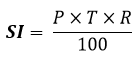## C program to calculate Simple Interest

Write a C program to input principle, time and rate (P, T, R) from user and find Simple Interest. How to calculate simple interest in C programming. Logic to find simple interest in C program.

#### Required knowledge

Operators, Data Types in c, Variables in C, Basic input/output

#### Simple Interest formula

Simple interest formula is given by.Where,

P is the principle amount

T is the time and

R is the rate

#### Logic to calculate simple interest

Step by step descriptive logic to calculate simple interest.

• 1.Input principle amount in some variable say principle.

• 2.Input time in some variable say time.

• 3.Input rate in some variable say rate.

• 4.Find simple interest using formula SI = (principle * time * rate) / 100.

• 5.Finally, print the resultant value of SI.

#### Program to calculate simple interest

```

/**
* C program to calculate simple interest
*/

#include <stdio.h>

int main()
{
float principle, time, rate, SI;

/* Input principle, rate and time */
printf("Enter principle (amount): ");
scanf("%f", &principle);

printf("Enter time: ");
scanf("%f", &time);

printf("Enter rate: ");
scanf("%f", &rate);

/* Calculate simple interest */
SI = (principle * time * rate) / 100;

/* Print the resultant value of SI */
printf("Simple Interest = %f", SI);

return 0;
}
```

##### Output:
```
Enter principle (amount): 1200
Enter time: 2
Enter rate: 5.4
Simple Interest = 129.600006
```
```
Recommended posts

Loop programming exercises index.
Basic programming exercises index.
If else programming exercises index.
Array programming exercises index.
String programming exercises index.
Pointer programming exercises index.
Star Patterns exercises index.
Number Patterns exercises index.
alphabet Patterns exercises index.
series program exercises index.

```